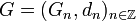# Chain complex of groups

(diff) ← Older revision | Latest revision (diff) | Newer revision → (diff)

## Definition

### Over the integers

A chain complex of groups is defined as the following data:

• A collection$G_n$ of groups, where$n$ varies over the integers
• A collection$d_n:G_n \to G_{n-1}$ of group homomorphisms, called the differentials.

such that for any$n$:$d_{n-1} \circ d_n = 0$

The chain complex is typically written as:$\dots \to G_n \stackrel{d_n}{\to} G_{n-1} \stackrel{d_{n-1}}{\to} G_{n-2} \to \dots$

### Over a contiguous segment of the integers

Suppose$a \le b$ are integers. A chain complex with index from$b$ to$a$ is a chain complex of groups in the above sense (over all integers) but with$G_n$ the trivial group for$n < a$ or$n > b$. This kind of chain complex is usually written in shorthand as:$1 \to G_b \to G_{b-1} \to \dots \to G_{a+1} \to G_a \to 1$

where 1 denotes the trivial group.

### Abstract definition

A chain complex of groups is a group object in the category of chain complexes of pointed sets.

## Homomorphism of chain complexes

There are many notions of homomorphism of chain complexes, each discussed below. Each notion of homomorphism defines a corresponding notion of isomorphism.

### Homomorphism that preserves the positions

Given two chain complexes$G = (G_n,d_n)_{n \in \mathbb{Z}}$ and$H = (H_n,\partial_n)_{n \in \mathbb{Z}}$ of groups, a homomorphism$\varphi:G \to H$, also called a chain map or degree zero chain map, is a collection of group homomorphisms:$\varphi_n:G_n \to H_n$

such that, for all$n$:$\partial_n \circ \varphi_n = \varphi_{n-1} \circ d_n$

where both sides describe group homomorphisms from$G_n$ to$H_{n-1}$.

### Homomorphism that allows for a shifting of positions

Let$m$ be an integer. Given two chain complexes$G = (G_n,d_n)_{n \in \mathbb{Z}}$ and$H = (H_n,\partial_n)_{n \in \mathbb{Z}}$ of groups,$\varphi:G \to H$, a homomorphism of degree$m$, also called a degree$m$ chain map, if it is a collection of group homomorphisms:$\varphi_n:G_n \to H_{n+m}$

such that, for all$n$:$\partial_{n+m} \circ \varphi_n = \varphi_{n-1} \circ d_n$.

## Terminology

• If the image of$d_n$ equals the kernel of$d_{n-1}$, then the chain complex is said to be exact at$G_{n-1}$. If this is true for every$n$, the chain complex is termed an exact sequence of groups.
• If the image of$d_n$ is a normal subgroup of the kernel of$d_{n-1}$, the quotient group is termed the$(n-1)^{th}$ homology group of the chain complex.
• If the image of$d_n$ is a normal subgroup of the whole next group$G_{n-1}$, then the preceding condition holds and we can define the homology. A chain complex of groups where this holds for all$n$ is termed a normal complex of groups.